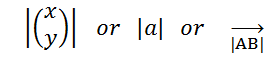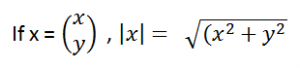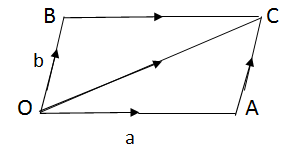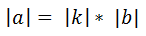Thursday , August 6 2020
ONLINE BOOK STORE (Shop Now)
Home / Igcse Mathematics Revision Notes / Vectors (In Two Dimensions)

# Vectors (In Two Dimensions)

Vectors have both magnitude and direction.### Column Vector:### Resultant Vector:

Single Letter in bold. i.e  a.

### Position Vector:

Position vectors are also called as end points.### Magnitude:To Find the Modulus / Magnitude of a position vector:### Addition And Subtraction of Vectors:(Triangular Law of Addition) nose to tail

• The negative sign reverses the directionof a vector.
• The result of a-b is a + – b e. subtracting b is equal to adding the negative of b.

### Equal Vectors:

If two line segmants AB  and CD are parallel, in same direction and equal in length, then AB and CD are equal vectors.### Parallel Vectors:

Vectors are parallel, if they have the same direction.

Both components of one vector must be in the same ratio to the corresponding components of the parallel vector.

Vector b                        kb (k>0)                         kb (k<0)If a = kb, the vectors a and b are parallel and are in same direction.

Magnitude of a isRelax your Mind From Studying and WATCH this Beautiful Sunset Painting.

SUBSCRIBE to learn How to Paint